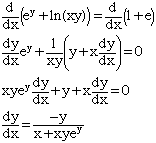student grade 12 calculus write an equation of the line tangent to the graph of ey + ln(xy) = 1 + e at (e,1) please help cant figure out Hi, I first checked to see if (e,1) satisfies the equation using substitution e1 + ln(e) = e + 1 Hence (e,1) is on the graph. Thus the tangent line is the line through (e,1) with slope given by the derivative of the expression at the point (e,1). You can find the derivative using implicit differentiation. Differentiating both sides of ey + ln(xy) = 1 + e I getCan you complete the problem now? Harley Go to Math Central